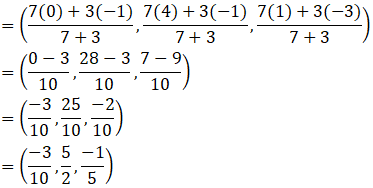A(1, 2, 3), B(0, 4, 1), C(-1, -1, -3) are the vertices of a triangle ABC. Find the point in which the bisector of the angle ∠BAC meets BC.

Asked by Sakshi | 1 year ago |  157

##### Solution :-

Given:

The vertices of a triangle are A (1, 2, 3), B (0, 4, 1), C (-1, -1, -3)

By using the distance formula,

The distance between A(1, 2, 3) and B (0, 4, 1) is AB

$$\sqrt{(1-0)^2+(2-4)^2+(3-1)^2}$$

$$\sqrt{9}=3$$

The distance between A(1, 2, 3) and C(-1, -1, -3) is AC

$$\sqrt{(1-(-1))^2+(2-(-1))^2+(3-(-3))^2}$$

$$\sqrt{49}=7$$

So, $$\dfrac{AB}{AC}$$ = $$\dfrac{3}{7}$$

AB: AC = 3:7

BD: DC = 3:7

Then, m = 3 and n = 7

B(0, 4, 1) and C(-1, -1, -3)

Coordinates of D by using section formula is given asThe coordinates of D are ($$\dfrac{-3}{10}$$, $$\dfrac{5}{2}$$, $$\dfrac{-1}{5}$$).

Answered by Aaryan | 1 year ago

### Related Questions

#### The mid-points of the sides of a triangle ABC are given by (-2, 3, 5), (4, -1, 7) and (6, 5, 3). Find the coordinates

The mid-points of the sides of a triangle ABC are given by (-2, 3, 5), (4, -1, 7) and (6, 5, 3). Find the coordinates of A, B and C.

#### If the points A(3, 2, -4), B(9, 8, -10) and C(5, 4, -6) are collinear, find the ratio in which C divided AB.

If the points A(3, 2, -4), B(9, 8, -10) and C(5, 4, -6) are collinear, find the ratio in which C divided AB.

#### Find the ratio in which the line segment joining the points (2, -1, 3) and (-1, 2, 1) is divided by the plane

Find the ratio in which the line segment joining the points (2, -1, 3) and (-1, 2, 1) is divided by the plane x + y + z = 5.